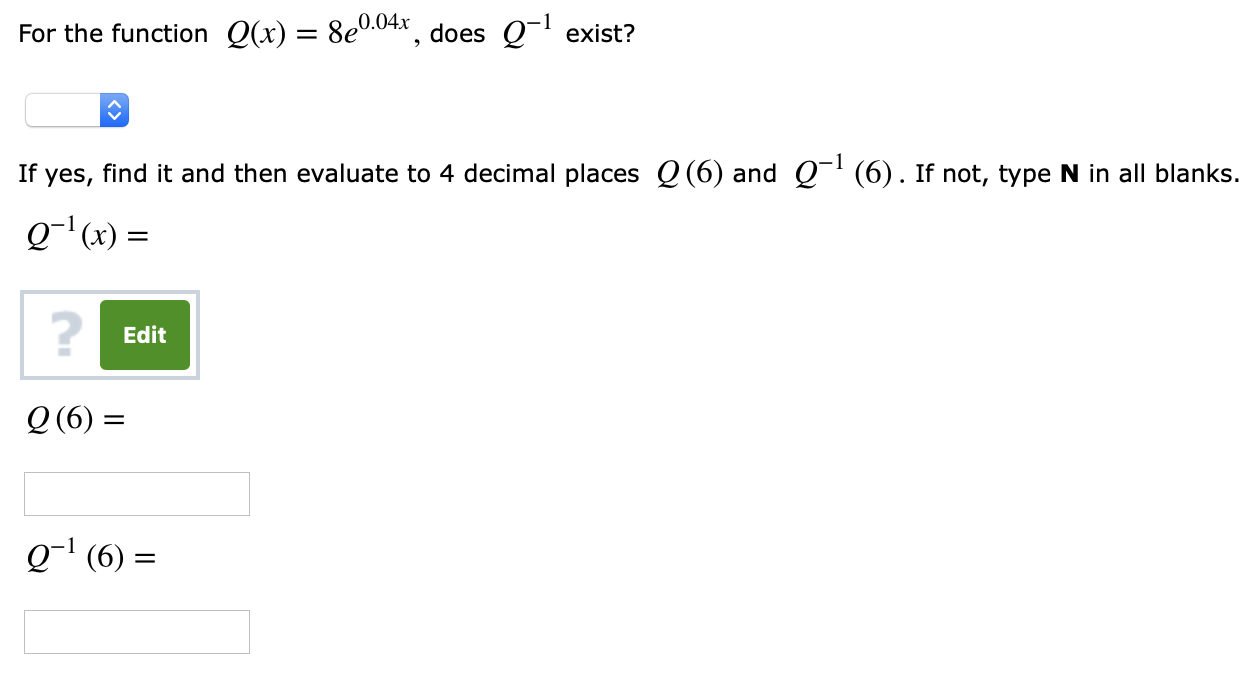For the function Q(x) = 8e004. does Q-i exist?If yes, find it and then evaluate to 4 decimal places Q(6) and Q1 (6). If not, type N in all blanks.Q-1 (x) =2EditQ (6) =01 (6)

Questionhelp_outlineImage TranscriptioncloseFor the function Q(x) = 8e004. does Q-i exist? If yes, find it and then evaluate to 4 decimal places Q(6) and Q1 (6). If not, type N in all blanks. Q-1 (x) = 2 Edit Q (6) = 01 (6) fullscreen
Step 1

Calculation:

The given function is Q(x) = 8e0.04x and the given point is x = 6.

Substitute x = 6 in the function Q(x) = 8e0.04x as follows.

Step 2

Replace Q(x) = 8e0.04x by y as y = 8e0.04x.

Interchange the variables and solve for y.

Step 3

Further simplif...

Want to see the full answer?

See Solution

Want to see this answer and more?

Our solutions are written by experts, many with advanced degrees, and available 24/7

See Solution
Tagged in

Algebra